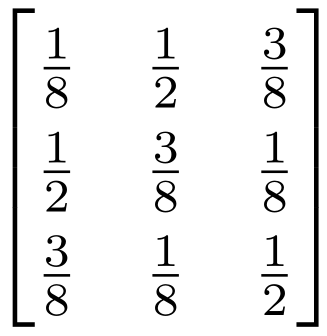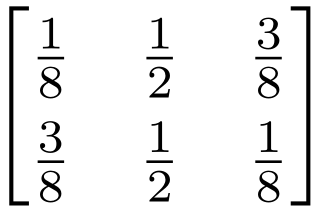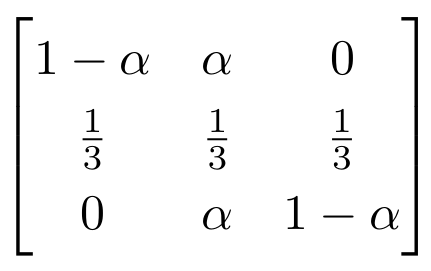# 第三章 信道及其容量--离散无记忆信道的容量【含答案】 应用信息论基础

（1）单选题A  对称信道

B  弱对称信道，但不是对称信道

C  以上说法都不对

（2）单选题A  对称信道

B  弱对称信道，但不是对称信道

C  以上说法都不对

（3）单选题A  对称信道

B  弱对称信道，但不是对称信道

C  以上说法都不对

（4）判断题

（5）单选题

A  p(X = 2) = 0

B  0 < p(X = 2) < 1

C  p(X = 2) = 1

（6）单选题

A  p(X = 2) = 0

B  0 < p(X = 2) < 1

C  p(X = 2) = 1